This applet demonstrates distances across the surface of a rectangular box. More information below.

Since you are reading this, you are not seeing the applet. Sorry, but I hope the rest of the page makes some kind of sense.

Contents

Applet How to use Commentary Surface of a cube
Spider and Fly Ant Square prism Surface Distance Conjecture General cuboid

How to use: ContentsApplet

The first three boxes are the dimensions of the cuboid. To change them, type new numbers in and then press the "You choose" button. Numbers between about 1 and perhaps 50 seem to work best, but you can try others higher or lower. The pictures are not perfect, due to rounding effects.

The text box offers six almost self-explanatory choices for the picture.

With "distance from clicked point", just click a point on the cuboid, and the picture will show distances on the surface starting from that point. A small black x (or perhaps more than one) should appear at the point furthest from the starting point.

With "sides used from starting point", the picture tries to show (by using a variety of colours) the faces used in shortest route from the clicked starting point to every other point on the cuboid. It takes a little getting used to; in particular, all points on the same face as the starting point only require routes using that face. Again, a small black x (or perhaps more than one) should appear at the point furthest from the starting point, and a dot should appear at the starting point.

With " number of sides from clicked point", the picture tries to show (by using a variety of colours) the number of faces used in shortest route from the clicked starting point to every other point on the cuboid. The only possibilities are 1 (i.e. to points on the same face), 2, 3, 4, or 5 (which if it occurs will be on the opposite face - this counterintuitive case involves a route using all but one of the cuboid's faces and was first mentioned by Dudeney).

With "distance to opposite point", the picture shows for each point the surface distance of the point on the cuboid which is opposite that point through the centre of the cuboid. Here a small black x indicates a point on the cuboid for which this is maximised.

With " number of sides to opposite point", the picture again tries to show (by using a variety of colours) the number of faces used in shortest route from the clicked starting point to every other point on the cuboid. The only possibilities are 3, 4, or 5 (this counterintuitive case is related to the Dudeney Spider and Fly problem below).

With "distance to furthest point", the picture shows for each point the surface distance of the point furthest on the surface from that point. Here a small black x indicates a point on the cuboid for which this is maximised. THIS IS VERY SLOW: theoretically the time should depend roughly on the 4th power of the screen scale (i.e. the square of the screen area) of the picture of the cuboid, so before selecting this choice it might be sensible to reduce the scale using the next function (perhaps to 60?) before selecting this choice, and if this choice works fast enough, try increasing the value slowly until an acceptable time/detail balance is achieved. The relative values of the three dimensions will also have an impact, as the speed is inversely proportional to the square of the screen area used.

The final function affects the scale of the picture on the screen and can be reduced to improve speed or increased to improved detail.

The picture does not work well in 256 colours, and a higher number improves the position. Each sharp transition from red to yellow is supposed to signify a change of unit in the distance being shown, with a slow build up from yellow though orange to red showing increasing distances in fractions of a unit. More red/yellow transitions can be introduced by multiplying all three dimensions by a common number, and this may be particularly useful when looking at some choices for the picture (e.g. looking at a cube, the first choice are meaningful for 1x1x1, the second and third and fifth do not really depend on the overall scale, while the fourth and sixth look much better with 10x10x10 or more).

Commentary ContentsApplet

This work has been stimulated by three sources available on the web:

To add to the curiosities that this problem produces, here is another simpler (and I think therefore odder) one:

Find a point on a cube which is the furthest on the surface from the mid-point of an edge. (Hint: the intuitively obvious possibilities seem to be a corner of the cube, or the mid-point of the opposite edge - neither are correct) Solution

Any other sources of similar questions would be welcome.

Cube ContentsApplet

The following assertions seem plausible, based on a combination of experimentation and calculation:

If a point P on a cube of side s is on face F with the nearest corner at C and is distance x from the nearest edge D and is distance y from the next nearest side E where D and E are edges of F which meet at C (i.e. 0 <= x <= y <= s/2), with OC being the corner opposite C through the centre of the cuboid, OD and OE similarly being the edges opposite D and E respectively and OF being the face opposite F, then:

1. The point GP which is the furthest on the surface from P is on the face OF opposite F;
2. GP is X from the side OD opposite D and Y from the side OE opposite E where:
X = x and Y = Max( (2x(s-x)+ys)/(3s-2y) , (s-x)(x+y)/(2s+x-y) ) where x <= Y <= y;
3. if y = s/2 then this gives X = x and Y = Max( x(s-x)/s+s/4 , (s-x)(2x+s)/(3s+2x) ),
but there is a second solution with X = x and Y = Min( ( 3s/4-x(s-x)/s , (2x2+xs+2s2)/(3s+2x) ) where s/2 <= Y <= s-x;
4. if x = 0 and y = s/2 then the two solutions have X = 0 and Y = s/3 or Y = 2s/3,
i.e. the points on a cube which are the furthest on the surface from the mid-point of an edge are a third and two-thirds of the way across the opposite edge; the following picture aims to shows the four possible routes to each of the two points at greatest distance from the mid-point of an edge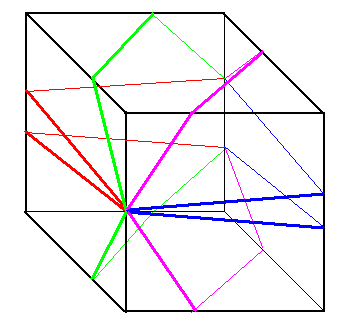5. in general, the surface distance from P to GP is sqrt(4s2+(s-y-Y)2) with Y = Max( (2x(s-x)+ys)/(3s-2y) , (s-x)(x+y)/(2s+x-y) );
6. sqrt(4s2+(s-y-Y)2) is maximised when y = Y = 0 (requiring x = 0),
and minimised when y = Y = s/2 (requiring x = s/2) at a value of 2s ;
so on a cube, the pairs of greatest surface distance points which maximise the greatest distance are opposite corners, while the pairs which minimise the greatest distance are mid-points of opposite faces;
7. the surface distance from P to the point OP opposite P through the centre is sqrt(4s2+(s-2y)2 which does not depend on x apart from the constraint 0 <= x <= y <= s/2;
8. iff x = y, i.e. P is on the diagonal of a face, then X = Y = x = y, i.e. GP is OP the opposite point to P through the centre of the cube;
9. the number of routes of shortest distance from P to GP depends on the position of P (i.e. on the values of x and y): if x=y=0 there are six routes; otherwise if x=0, if x=y, or if (2x(s-x)+ys)/(3s-2y)=(s-x)(x+y)/(2s+x-y) there are four routes; and in all other cases there are three routes; (if y=s/2 and x<s/2 then there are three (or in two cases, four) routes to each of the two possibilities for GP;)
10. for some values of x and y (with max(sqrt(2-x2)-1,(sqrt(3-4x2+4x)-1)/2)<y<1/2, i.e. with y close to 1/2), there is a point MP on OF which is a local maximum surface distance from P, although it is closer to P than GP is;
11. the point on an adjacent face which is furthest from P is on OD a distance Z = s(y-x)/(2s-x-y) from C and the surface distance from P is sqrt((2s-x)2+(s-y-Z)2);
• if y=x then this point is OC and Z = 0;
• if x=0 then this point is GP and Z = sy/(2s-y);
• if y=s/2 then Z = (s2-2xs)/(3s-2x), but there is a second solution with Z = 2s2/(3s-2x);
• if y=x=s/2 then the points sought are the four corners of the face OF.

The following graph indicates how the formula for the co-ordinates of the point at greatest surface distance depends on the values of x and y. In the light green near the edge Y=(s-x)(x+y)/(2s+x-y), while in the light blue near the centre Y=(2x(s-x)+ys)/(3s-2y).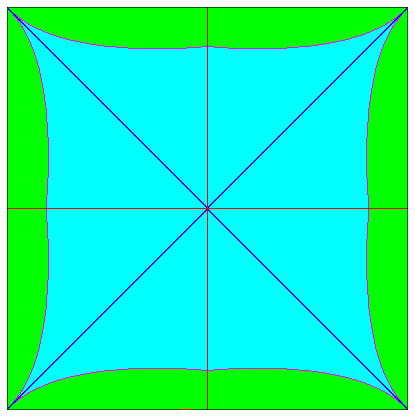The following picture of a cube aims to illustrate the four routes of shortest distance from a point between the light blue and light green areas above, for example x=s/10, y=3s/10 (in which case Y=2s/10). For a point closer to the edge (i.e. in the light green area) the light green route would be shorter than the light blue route, while for a point further from the edge (i.e. in the light blue area) the light blue route would be shorter than the light green route.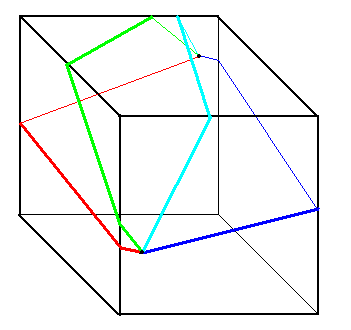To describe the points with different properties, we clearly only need to consider an eighth of a face, using symmetry to cover the rest of the face and of the cube. The following picture labels some of the interesting points on a 1x1x1 cube, and these are described in more detail below: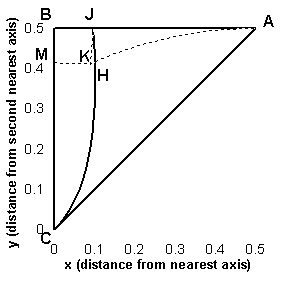We can then say:

• A (0.5,0.5) or (1/2,1/2): centre of face: furthest point is centre of opposite face with four shortest routes; also represents smallest distance a point and its furthest point.
• B (0,0.5) or (0,1/2): centre of edge: two furthest points each on the opposite edge a third of the way alonfg that edge from a corner, with four shortest routes to each point.
• C (0,0): corner: furthest point is opposite corner, with six shortest routes, also represents greatest distance between two points.
• J (0.096968283237315,0.5) or ((1-8*Cos(Pi/3+aCos(5/32)/3))/6,1/2): Other point (in addition to B) with four shortest routes to two points at greatest distance
• On AB excluding A: y=1/2 and 0<x<1/2: points with two furthest points, with three (apart from point J which has four) shortest routes to each
• On BC (i.e. edge) excluding B and C: x=0 and 0<y<1/2: furthest point is on opposite edge with four shortest routes
• On AC (i.e. face diagonal) excluding A and C: 0<x=y<1/2: furthest point is opposite point with four shortest routes
• H (0.101910213188,0.37200096992612) or ((1+sqrt(5)-sqrt(8))/4,1-1/(1-sqrt(5)+sqrt(8))): non-face-diagonal (i.e. apart from point A and points on AC) point with four shortest routes to point of greatest distance which has highest value of x .
• On CHJ excluding C and J: y=1-(1+/-sqrt(1-12x+20x2+16x3-16x4))/(2-4x) with 0<y<1/2 :other points with four shortest routes to point of greatest distance (in addition to A, J, B, points on AC and points on BC. Note that on AH +/- is positive, while on HJ +/- is negative.
• In AHJC (strictly between AC, AJ and CHJ, i.e. the light blue area above) and in BCHJ (strictly between BC, BJ and CHJ, i.e. the light green area above) there are three shortest routes to the point of greatest distance.

We can also consider those points where there is a local maximum:

• M (0,0.41421356237309) or (0,sqrt(2)-1): limit from above of points with x=0 which have a point which is a local maximum distance away but not a global maximum. (M itself does not have the property)
• On BM excluding B and M: x=0 and sqrt(2)-1<y<1/2: points with x=0 which have a point which is a local maximum distance away but not a global maximum, with four shortest routes to this local maximum distance point
• K (0.088562172233852,0.41143782776614) or ((3-sqrt(7))/4,(sqrt(7)-1)/4): other limit (apart from M) from above of points which have four shortest routes to a point which is a local maximum distance away but not a global maximum. (K itself does not have the property)
• On JK excluding J and K: y=(1-sqrt(1-4x*(1-2x)*(1+x)*(3-2x)))/(2-4x) and (3-sqrt(7))/4<x<(1-8*Cos(Pi/3+aCos(5/32)/3))/6: other points (apart from those on BM) which have four shortest routes to a point which is a local maximum distance away but not a global maximum. Note that JK is effectively the extension of AHJ after reflection in AJB.
• In AJBMK (strictly between AJB and AKM): max(sqrt(2-x2)-1,(sqrt(3-4x2+4x)-1)/2)<y<1/2 and 0<=x<1/2: points which have a point which is a local maximum distance away but not a global maximum, with three (apart from points on BM or JK, which have four) shortest routes to this local maximum distance point. Note that AK has the form y=(sqrt(3-4x2+4x)-1)/2 and (3-sqrt(7))/4<=x<1/2; and that KM has the form y=sqrt(2-x2)-1 and 0<=x<=(3-sqrt(7))/4.

The following picture shows the surface distance to the point of greatest surface distance from each point on a 20x20x20 cube, taken from the java applet above. The distances involved are 40 at the centre of each square increasing to sqrt(2000)=44.721... at the corners.By comparison, the next picture shows the surface distance to the opposite point from each point on a 20x20x20 cube, taken from the java applet above. Since the point of greatest distance form the centre of a face or a corner (or indeed any point on a diagonal of a face) is the opposite point, the range of distances involved is again 40 to sqrt(2000)=44.721...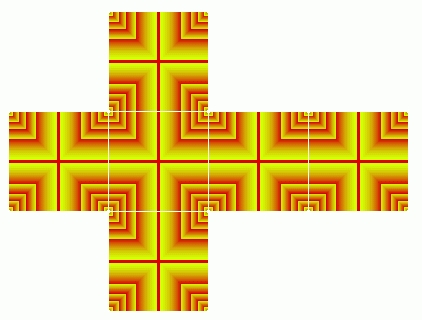Many more questions could be asked. For example, we could look for closed routes which cross or visit each edge or face of a cube. Some suggested answers can be found on the page Circumnavigating a cube and a tetrahedron.

Cuboid ContentsApplet

Of the assertions for a cube, none apply directly to all points P on all cuboids apart from the fact that there are cases of more than one point at greatest surface distance from some points P and there are points which are local maximum surface distances though not greatest surface distances from some other points P.

Spider and Fly Problem ContentsApplet

Henry Ernest Dudeney's Spider and Fly Problem: With a cuboid 30x12x12, what is the minimum surface distance from a point which is on a 12x12 face and in 1 from the mid-point of an edge to the opposite point across the centre of the cuboid?

This is just a matter of checking all the possibilities: the Java applet above looks at twenty, but it is clear that for this special case we will only consider four plausible shortest routes (the distance of each in fact represents more than one possibility because of the symmetries in the question).

• One (a three face solution) is to go to the nearest edge, then across the 30x12 face and finally to the opposite point for a total distance of 1+30+11=42.
• Another (also a three face solution) is to go to an edge next to the nearest edge, then across the 30x12 face and finally to the opposite point for a total distance of sqrt(102+(6+30+6)2)=sqrt(1864)=43.174...
• Another (a four face solution) is to go to the nearest edge, then cut across a corner of the 30x12 face, then cut across another corner of the next 30x12 face, and finally go to the opposite point, for a distance of sqrt((6+11)2+(1+30+6)2)=sqrt(1658)=40.718...
• Finally (a five face solution) we can go to the nearest edge, then cut across the corner of the 30x12 face, then to the opposite side of the next 30x12 face, then cut across the corner of the next 30x12 face and finally go to the opposite point, for a distance of sqrt((6+12+6)2+(1+30+1)2)=sqrt(1600)=40.

The five face solution (or its reflection) clearly results in the shortest surface distance and 40 answers the question. Dudeney chose his problem to keep most of the numbers as integers and to produce a (not immediately obvious) five face solution.

Further comments on the spider and the fly problem ContentsApplet

The furthest point from the starting point is in fact in this case where the four face and five face solutions give equal distances: if it is x in from the mid-point of the nearest edge then sqrt((6+12-x)2+(1+30+6)2)=sqrt((6+12+6)2+(1+30+x)2) i.e. when x=156/98=1.5918... and the surface distance from the starting point is 40.475...

Many (but not all) of the points on the 30x12 faces have their point of greatest distance on an adjacent 12x12 face (not surprisingly the further one). For an XxXxY cuboid where Y/X>=(sqrt(17)+3)/2=3.56155..., all points on one of the four largest faces have points of greatest distance on an adjoining small face. Indeed for a WxXxY cuboid where X>=W and Y>=max[X+W/2+sqrt(X2+3XW+W2/4),X2/W+2X], all points of greatest distance from any starting point on the cuboid are on one or other of the two smallest (WxX) faces. The first value (in max[]) applies if 1<=X/W<=(cbrt(37+sqrt(1026))+cbrt(37-sqrt(1026))-2)/3=1.26953..., and requires a cuboid twice the length (Y) of one where the point furthest from a corner is not the opposite corner (see below). The second value (in max[]) applies if X/W>=(cbrt(37+sqrt(1026))+cbrt(37-sqrt(1026))-2)/3=1.26953..., and requires a cuboid twice the length (Y) of one with X>=W and Y>X2/2W+X , which then has the property that the surface distance between opposite corners is less than the surface distance between the centres of the WxX faces (though neither represents the maximum greatest surface distance on the cuboid).

For a 30x12x12 cuboid, there are four pairs of points which have the greatest surface distance between the points in the pair; each point is on one of the 12x12 faces, a distance sqrt(189)-9=4.7477... in from two adjacent edges (i.e. on a diagonal of the face), with the surface distance between them being sqrt(3420-sqrt(2721600))=42.0746... and each such point being the opposite point through the centre of its paired point. They occur where two three face and two four face solutions give the same distance. This distance maximal surface distance compares with the surface distance between the centres of the 12x12 faces of 42 and between opposite corners of sqrt(1476)=38.4187... (though, as we shall see in the ant problem below, that the furthest point from a corner is not in this case another corner).

Ant Problem ContentsApplet

Yoshiyuki Kotani and Donald Knuth's Ant Problem: With a cuboid 1x1x2, what is the furthest point on the surface from a corner (Kotani), and which are the pairs of points with the greatest surface distance between them (Knuth)?

The solutions to these two problems are similar to the those answered in the further comments on the Spider and Fly problem.

The point with greatest surface distance from a given corner is on the further 1x1 face and is a and b in from the sides where of the four expressions: sqrt((3-a)2+(1-b)2), sqrt((3-b)2+(1-a)2), sqrt((2-a)2+(2+b)2), and sqrt((2-b)2+(2+a)2), three are equal and the remaining one is greater than or equal to the others. This gives the solution that the point of greatest distance from a given corner of a 1x1x2 cuboid is on the 1x1 face and 1/4 in from each of the two sides of that face which meet at the opposite corner to the starting corner. The distance involved is sqrt(65/8)=2.8504... less than 1% more than sqrt(8)=2.8284... for the distance corner to corner. This result is easily generalised to any square prism or to any cuboid.

This result is easily checked in the Java applet above, particularly if the dimensions used are 4x4x8 and you then click on the little x which appears, seeing that the distance of that point is then 1 from the two nearest edges.

An equivalent question was posed in New Scientist in December 1998, leading to a usenet discussion. Many wrong answers were suggested. Even the relatively simple question of the shortest surface distance between opposite corners of a 2x1x1 cuboid posed around the same time also produced wrong answers.

The second part of the ant problem is essentially the same as that of the similar question of the spider and fly cuboid, with the result being four pairs of points which have the greatest surface distance between the points in the pair; each point is on one of the 1x1 faces, a distance (sqrt(3)-1)/2=0.3660... in from two adjacent edges (i.e. on a diagonal of the face), and each such point being the opposite point through the centre of its paired point. The surface distance between each pair is sqrt(16-sqrt(48))=3.0119...., marginally more than 3 which is the distance between the centres of the 1x1 faces.

Further comments on the ant problem: generalising for a square prism ContentsApplet

Having seen that the cuboids in the ant problem and in the spider and fly problem have many features in common, the question is whether we can produce a general response to the questions in the ant problem for all cuboids of the form AxAxB, i.e. for square prisms. The following assertions describe that generalised result:

1. If B/A<=1 then the greatest surface distance is represented by the four pairs of opposite corners and the distance involved is sqrt(2A2+2AB+B2), and so the point at greatest distance from a corner is the opposite corner;
2. If 1<=B/A<(sqrt(17)+3)/4=1.7807... then the point at greatest distance from a corner is the opposite corner at a distance of sqrt(4A2+B2);
3. If B/A>(sqrt(17)+3)/4=1.7807... then the point at greatest distance from a corner is on the further AxA face at a distance A/2-A2/2B in from the edges that meet at the opposite corner, at a surface distance of sqrt(B2+AB+3A2/2+A3/B+A4/2B2) from the starting point ;
4. If B/A=(sqrt(17)+3)/4=1.7807... then both the opposite corner and the point (sqrt(17)-1)A/2(sqrt(17)+3)=0.2192...A in from the relevant edges are at greatest surface distance of (sqrt(153)+13)A/8=7.1711...*A from the starting corner;
5. If 1<=B/A<sqrt(2)=1.4142... then the greatest surface distance is represented by the four pairs of opposite corners and the distance involved is sqrt(4A2+B2);
6. If B/A>sqrt(2)=1.4142... then the greatest surface distance is represented by four pairs of opposite points where each point is on an AxA face and is (sqrt(B2-A2)-B+A)/2 in from two edges, and where the distance involved is sqrt(3B2+2AB-2Bsqrt(B2-A2));
7. If B/A=sqrt(2)=1.4142... then the greatest surface distance is represented by eight pairs of opposite points being either pairs of opposite corners or pairs of opposite points where each point is on an AxA face and is (1-sqrt(1/2))A=0.2928...A in from two edges, and where the distance involved is sqrt(6)A=2.4394...A.

Surface Distance Conjecture ContentsApplet

Jim Propp's Surface Distance Conjecture: For a centrally symmetric convex compact body, the greatest surface distance between two points is achieved only for pairs which are opposites through the centre.

Little of what has been said so far can be generalised to all centrally symmetric convex compact bodies, but experimentation with the applet above suggests something which would, if true, imply the conjecture. The following statement may be stronger than what might be required to prove the conjecture, though it might point in a useful direction:

For a centrally symmetric convex compact body, if a point G of greatest surface distance from a starting point P is not opposite P through the centre of the body, then P is not a point of greatest surface distance from G.

This would imply that there could be no non-opposite pair of points P and G each of greatest surface distance starting from the other, and therefore there could be no non-opposite pair of points with the greatest surface distance between them of any two points on the body.

Note that the following related statement is obviously true by the central symmetry of the body:

For a centrally symmetric body, if a point G of greatest surface distance from a starting point P is the symmetric point to P (i.e. G is opposite P through the centre of the body and the internal distances from P and from G are the same), then P is a point of greatest surface distance from G.

Meanwhile the following is frequently not true even for a cube:

If two opposite points P and G are each of greatest surface distance starting from the other, then this surface distance is the greatest possible of any two points on the body.

But I suspect none of these comments add much to the solution of the problem. Even proving the conjecture for a cuboid requires a proper proof of the following assertions which attempt (not very well) to generalise the issue of the pairs of points at greatest distance.

Points of greatest surface distance from a corner ContentsApplet

The first of the ant questions is easily generalised for an AxBxC cuboid with A<=B<=C:

1. If B>C(2C-A)/(2C+A) [or equivalently A<2C(C-B)/(C+B) or C>(2B+A+sqrt(4B2+12AB+A2))/4] then the point at greatest distance from a corner is the opposite corner, and the distance involved is sqrt(A2+B2+C2+2AB);
2. If B<C(2C-A)/(2C+A) then the point at greatest distance from a corner is on the further AxB face at a distance A/2-AB/2C in from each of the edges that meet at the opposite corner, and the distance involved is sqrt((1+(B/C)2)(C2+AC+A2/2)) from the starting point ;
3. If B=C(2C-A)/(2C+A) then there are two points at greatest distance from a corner, which are the opposite corner and the point on the further AxB face at a distance A/2-AB/2C in from each of the edges that meet at the opposite corner, and the distance involved is sqrt(A2+B2+C2+2AB) from the starting point. Curiously, when there are two points at greatest distance from a corner, if A and C are rational, or if B and C are rational, then all three sides must be rational; however if A and B are rational, then C can be (and often is) irrational.

The numerical examples below describe some interesting integer cases while the Javascript calculator provides an easy way of applying these results.

Pairs of points of greatest surface distance on a cuboid ContentsApplet

For an AxBxC cuboid with A<=B<=C:

1. if A=B=C then the points at greatest surface distance are the four pairs of opposite corners;
2. if B<(Csqrt(C4+2AC3+A2C2-2A3C-A4)-C3)/(C2-A2) then there is a solution for x (and thus y) in the equation sqrt(A2-2BC+2AC-4Bx+4x2)=(2Cx+AB-BC)/(C-B+2x)=A-2y [an unattractive quartic in x when multiplied out, though in this form and with the following range, it is easily solvable for x by successive interval bisection] with B/2-AB/2C<x<B/2-sqrt((A+B+2C)(B-A))/2, and so:
• if B<C-A+x+y then the greatest surface distance is represented by four pairs of opposite points where each point is on an AxB face and is x in from an edge length A and y in from an edge length B, and where the distance involved is sqrt(A2+B2+C2+2AC+4x2-4Bx);
• if B>C-A+x+y then the greatest surface distance is represented by the four pairs of opposite corners and the distance involved is sqrt(A2+B2+C2+2AB);
• if B=C-A+x+y then the greatest surface distance is represented by eight pairs of opposite points being either pairs of opposite corners or pairs of opposite points where each point is on an AxB face and is x in from an edge length A and y in from an edge length B, and the distance involved is sqrt(A2+B2+C2+2AB);
3. if B>=(Csqrt(C4+2AC3+A2C2-2A3C-A4)-C3)/(C2-A2) then:
• If B<(8C3+A3)/(8C2+4AC) then the greatest surface distance is represented by the four pairs of opposite corners, and the distance involved is sqrt(A2+B2+C2+2AB);
• If B>(8C3+A3)/(8C2+4AC) then the greatest surface distance is represented by two pairs of opposite points each on the AxB face in (B+C-sqrt((B+C)2-A(A+2C-2B)))/2 from the mid points of the shortest edges, and the distance involved is sqrt(B2+3C2+2AB+2BC-2Csqrt((B+C)2-A(A+2C-2B)));
• If B=(8C3+A3)/(8C2+4AC) then the greatest surface distance is represented by the four pairs of opposite corners and the two pairs of opposite points each on the AxB face in (B+C-sqrt((B+C)2-A(A+2C-2B)))/2 from the mid points of the shortest edges, and the distance involved is sqrt(A2+B2+C2+2AB);
4. So there are cuboids with two, four, six or eight pairs of points representing the greatest surface distance, and in every case they are opposite points (as required by the Surface Distance Conjecture for a Cuboid). The numerical examples below describe some interesting integer cases while the Javascript calculator provides an easy way of applying these results.

The following graphs show how the pairs of points representing the greatest surface distance vary with the dimensions of the cuboid. They assume that one of the sides of the cuboid is length 1 (or pick a side and divide the length of the other sides by the length of that side. The yellow area represents the dimensions resulting in the four pairs of opposite corners representing the greatest surface distance; the green area represents the four pairs of points strictly inside the smallest faces; the grey area (and the dark grey lines between grey and green) represent the two pairs of points strictly inside the smallest faces; the red line between yellow and grey represents the combination of the four pairs of corners and the two pairs of points strictly inside the smallest faces; and the blue line between green and yellow represents the combination of the four pairs of corners and the four pairs of points strictly inside the smallest faces. The right hand graph is a detail of part of the left hand graph, one of the places where the three lines and three areas meet.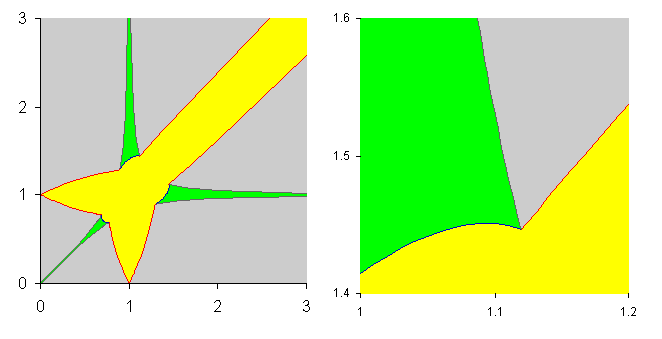Roughly in words, what this graph shows is that for the four pairs of opposite corners to represent the greatest surface distance (yellow), B must be close to C; B being A/2 away from C is too far, though this is approached as C increases relative to A. For four internal pairs to represent the greatest surface distance (green), B must be close to A and C must be significantly more; as C increases relative to A, the limit on the difference between B and A tightens quickly, although B can always equal A (a square prism). With reasonable gaps between A and B and between B and C, two internal pairs represent the greatest surface distance.

Numerical examplesContentsApplet

Many integer and other numerical examples are possible, in addition to those in the Spider and Fly Problem and the Ant Problem:

On a 6x6x6 cube, the two points at greatest distance from the mid-point of a side are on the opposite side 2 in from the corners; this is related to the fact that 145 is the sum of two squares in two ways: 122+12=92+82.

On a 10x10x10 cube the point at greatest distance from the point 1 in from the nearest side and 3 in from the next nearest side is almost opposite but is 1 in from a side and 2 in from another side; there are four routes from the starting point to the finishing point, and this is related to the fact that 425 is the sum of two squares in three ways: 202+52=192+82=162+132.

On an Nx(N-1)(N-2)/2xN(N-1)/2 cuboid with N>=5, for example a 5x6x10 or a 21x190x210 cuboid, the two points at greatest distance from a corner are the opposite corner and the point 1 in from each of the two edges on a smallest face which meet at the opposite corner; again this is related to numbers which are the sum of two different pairs of squares, since ((N2-N+2)/2)2+((N2-N)/2)2=((N2-3N)/2)2+((N2+N-2)/2)2, for example 221=112+102=142+52 and 88621=2112+2102=2302+1892.

By scaling the ant cuboid to 4x4x8, we get one of many cuboids with the point at greatest distance from a corner the point 1 in from each of the two edges on a smallest face which meet at the opposite corner; this is related to 130=92+72=112+32. Another square prism with the same property is 3x3x9 related to 125=102+52=112+22. There are numerous examples of other cuboids with the same property, such as 3x4x12, 3x5x15, 3x6x18, ..., 4x5x10, 4x6x12, 4x7x14, ..., 5x9x15, 5x12x20, 5x15x25, ..., 6x12x18, 6x14x21, 6x16x24, ..., etc. The pattern is based on the requirement for this property that B=C(A-2)/A as well as the basic constraint that B<C(2C-A)/(2C+A). We then have (B-1)2+(A+C-1)2=(A+B-1)2+(C+1)2.

The following examples demonstrate different possibilities for opposite points representing the greatest surface distance on the cuboid.

There are numerous examples of cuboids which have two pairs of opposite points representing the greatest surface distance on the cuboid which are the opposite pairs of points 1 in from the mid points of the shortest edges. One of the simplest is 4x5x10 with more of the form 4xNx(3N-2) with N>=5; others include 6x8x11 and others of the form 6xNx(2N-5) with N>=8, 8x16x21 and others of the form 8x2Nx(3N-3) with N>=8 etc.

The 3x3x5 cuboid has four pairs of opposite points representing the greatest surface distance on the cuboid, which are the points 1 in from two edges on a smallest face.

There are numerous examples of cuboids where the four pairs of opposite points representing the greatest surface distance on the cuboid are the corners of the cuboid: 1x1x1 is the simplest.

The 6x7x9 cuboid has six pairs of opposite points representing the greatest surface distance on the cuboid. They are the four pairs of opposite corners and the opposite pairs of points 1 in from the mid points of the shortest edges. The same is true for 8x13x16, 10x21x25, 12x31x36, ... and in general 2Nx(N^2-N+1)xN^2 with N>=3.

It is not clear whether there is a nice integer example of eight pairs of opposite points representing the greatest surface distance on the cuboid. A non-integer example is 1x1xsqrt(2), where four pairs are corners of the cuboid and the other four pairs are points (1-sqrt(1/2)) in from two edges on a smallest face. In the Javascript below the sides have to be 1, 1, and 1.414213562373 to achieve the desired result.

Javascript calculator (based on assertions above)ContentsApplet

Sides of the cuboid: